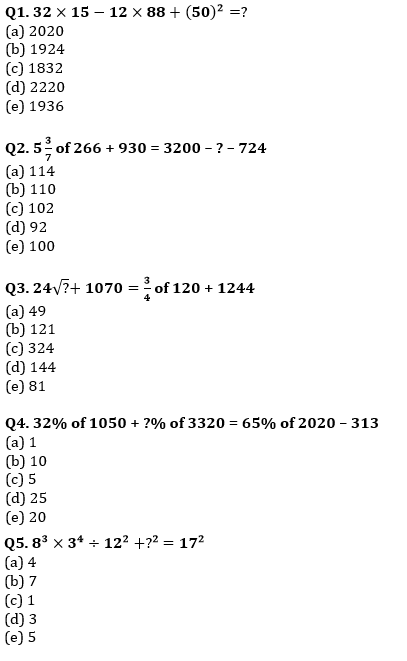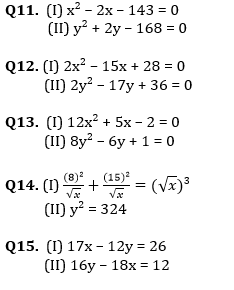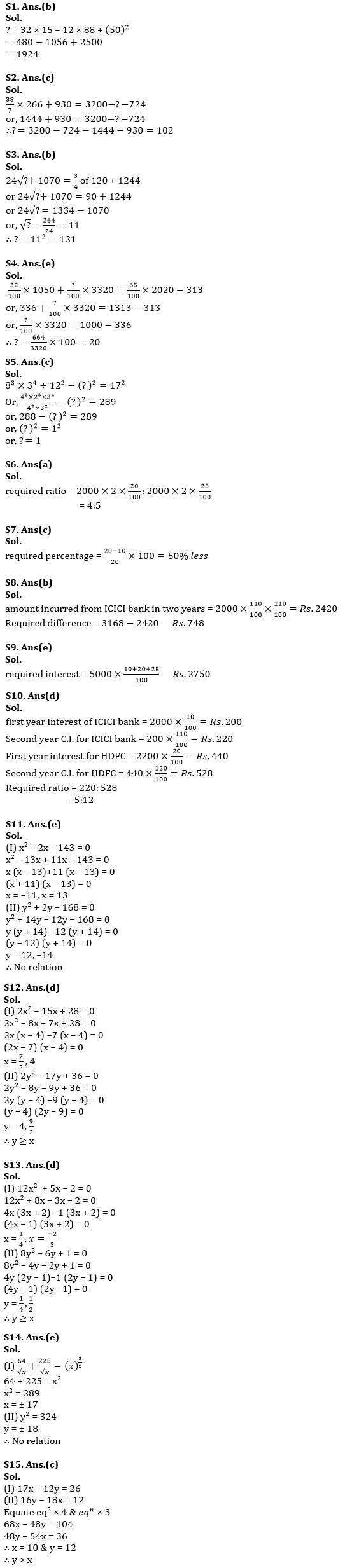Latest Banking jobs   »   Quantitative Aptitude Quiz For SBI Clerk...

# Quantitative Aptitude Quiz For SBI Clerk Prelims 2022- 29th May

Directions (1-5): What should come in place of question mark (?) in the following questions?Directions (6-10): Dharam invest some amount in ICICI bank at 10% per annum at C.I. for two years and get Rs. 420 as total interest. If he withdrew his sum one year after from ICICI and invested in HDFC bank at R% per annum at C.I for two years, the sum would become Rs.3168. Rate of interest for SBI is 25% more than that of HDFC.

Q6. If amount invested in HDFC or SBI bank is equal to amount invested in ICICI initially, find ratio of simple interest earned in two years for HDFC to S.I. earned for SBI in two years?
(a) 4:5
(b) 2:3
(c) 1:3
(d) 1:2
(e) None of these.

Q7. Rate of ICICI bank is how much percent more/less than rate of interest of HDFC bank?
(a) 50% more
(b) 25% more
(c) 50% less
(d) 25% less
(e) 20% less

Q8. What is the difference between total amount incurred in two year from HDFC bank and ICICI bank at C.I.?
(a) Rs. 608
(b) Rs. 748
(c) Rs. 760
(d) Rs. 560
(e) Rs. 768

Q9. If Rs. 5000 is invested for three years at rate of interest of ICICI, HDFC and SBI for 1st, 2nd and 3rd year respectively. Find total interest earned at simple interest?
(a) Rs. 1500
(b) Rs. 3000
(c) Rs. 2075
(d) Rs. 2725
(e) Rs. 2750

Q10. What is the ratio between second year C.I. of ICICI and HDFC bank?
(a) 5:11
(b) 7:13
(c) 2:5
(d) 5:12
(e) 12:13

Directions (11-15): In each of these questions, two equations (I) and (II) are given. You have to solve both the equations and answer the following questions.
(a) if x > y
(b) if x ≥ y
(c) if x < y
(d) if x ≤ y
(e) if x = y or no relation can be established between x and y.Solutions#### Congratulations!Union Budget 2023-24: Free PDF